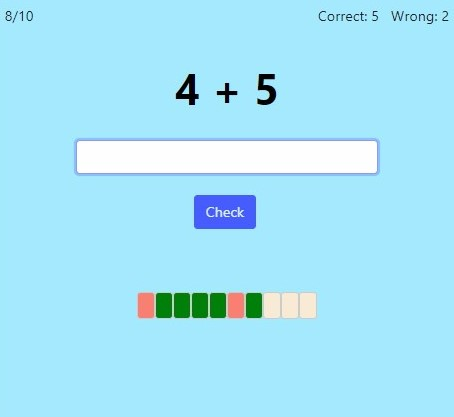Here you can practice the basic addition and subtraction facts using the fact families approach. This approach is used in the Math Mammoth curriculum (grade 1 and grade 2).

For example, if you choose to practice fact families with 6, you will get addition and subtraction facts from various fact families with the sum of 6:

0 + 6 = 6
6 + 0 = 6
6 − 0 = 6
6 − 6 = 0
1 + 5 = 6
5 + 1 = 6
6 − 1 = 5
6 − 5 = 1
2 + 4 = 6
4 + 2 = 6
6 − 2 = 4
6 − 4 = 2
3 + 3 = 6
3 + 3 = 6
6 − 3 = 3
6 − 3 = 3

Screenshot:You can choose which fact families to practice. The program will give you both addition and subtraction facts from those families, including missing number additions and subtractions.

For first grade, choose fact families through 10, and for second grade, choose, in particular, fact families from 11 through 18.

Additionally you can select timed or untimed practice and the number of practice problems.

OR
Practice for a set time: min